# matplotlib Plotting Cookbook5 (2 reviews total)
By Alexandre Devert

• Constantly updated with 100+ new titles each month
• Breadth and depth in over 1,000+ technologies

matplotlib is part of the Scientific Python modules collection. matplotlib provides a large library of customizable plots and a comprehensive set of backends. It tries to make easy things easy and hard things possible. You can generate plots, add dimensions to the plots, and also make the plots interactive with just a few lines of code with matplotlib. Also, matplotlib integrates well with all common GUI modules.

This book is a head-first, hands-on journey into matplotlib, the complete and definite plotting package for Python. You will learn about the basic plots, how to customize them, and combine them to make sophisticated figures. Along with basic plots, you will also learn to make professional scientific plots.

In this book, you will start with the common figures that are offered by most plotting packages. You will learn how to add annotations, and play with styles, colors, scales, and shapes so that you can add personality and visual punch to your graphics. You will also see how to combine several graphics. With this book you will learn how to create sophisticated visualizations with simple code. Finally, you can make your plots interactive.

After reading "matplotlib Plotting Cookbook", you will be able to create the highest quality plots.

Publication date:
March 2014
Publisher
Packt
Pages
222
ISBN
9781849513265

## Chapter 1. First Steps

In this chapter, we will cover:

• Installing matplotlib

• Plotting one curve

• Using NumPy

• Plotting multiple curves

• Plotting curves from file data

• Plotting points

• Plotting bar charts

• Plotting multiple bar charts

• Plotting stacked bar charts

• Plotting back-to-back bar charts

• Plotting pie charts

• Plotting histograms

• Plotting boxplots

• Plotting triangulations

## Introduction

matplotlib makes scientific plotting very straightforward. matplotlib is not the first attempt at making the plotting of graphs easy. What matplotlib brings is a modern solution to the balance between ease of use and power. matplotlib is a module for Python, a programming language. In this chapter, we will provide a quick overview of what using matplotlib feels like. Minimalistic recipes are used to introduce the principles matplotlib is built upon.

## Installing matplotlib

Before experimenting with matplotlib, you need to install it. Here we introduce some tips to get matplotlib up and running without too much trouble.

### How to do it...

We have three likely scenarios: you might be using Linux, OS X, or Windows.

Linux

Most Linux distributions have Python installed by default, and provide matplotlib in their standard package list. So all you have to do is use the package manager of your distribution to install matplotlib automatically. In addition to matplotlib, we highly recommend that you install NumPy, SciPy, and SymPy, as they are supposed to work together. The following list consists of commands to enable the default packages available in different versions of Linux:

• Ubuntu: The default Python packages are compiled for Python 2.7. In a command terminal, enter the following command:

```sudo apt-get install python-matplotlib python-numpy python-scipy python-sympy
```
• ArchLinux: The default Python packages are compiled for Python 3. In a command terminal, enter the following command:

```sudo pacman -S python-matplotlib python-numpy python-scipy python-sympy
```

If you prefer using Python 2.7, replace `python` by `python2` in the package names

• Fedora: The default Python packages are compiled for Python 2.7. In a command terminal, enter the following command:

```sudo yum install python-matplotlib numpy scipy sympy
```

### Note

There are other ways to install these packages; in this chapter, we propose the most simple and seamless ways to do it.

Windows and OS X

Windows and OS X do not have a standard package system for software installation. We have two options—using a ready-made self-installing package or compiling matplotlib from the code source. The second option involves much more work; it is worth the effort to have the latest, bleeding edge version of matplotlib installed. Therefore, in most cases, using a ready-made package is a more pragmatic choice.

You have several choices for ready-made packages: Anaconda, Enthought Canopy, Algorete Loopy, and more! All these packages provide Python, SciPy, NumPy, matplotlib, and more (a text editor and fancy interactive shells) in one go. Indeed, all these systems install their own package manager and from there you install/uninstall additional packages as you would do on a typical Linux distribution. For the sake of brevity, we will provide instructions only for Enthought Canopy. All the other systems have extensive documentation online, so installing them should not be too much of a problem.

So, let's install Enthought Canopy by performing the following steps:

1. Download the Enthought Canopy installer from https://www.enthought.com/products/canopy. You can choose the free Express edition. The website can guess your operating system and propose the right installer for you.

2. Run the Enthought Canopy installer. You do not need to be an administrator to install the package if you do not want to share the installed software with other users.

3. When installing, just click on Next to keep the defaults. You can find additional information about the installation process at http://docs.enthought.com/canopy/quick-start.html.

That's it! You will have Python 2.7, NumPy, SciPy, and matplotlib installed and ready to run.

## Plotting one curve

The initial example of Hello World! for a plotting software is often about showing a simple curve. We will keep up with that tradition. It will also give you a rough idea about how matplotlib works.

You need to have Python (either v2.7 or v3) and matplotlib installed. You also need to have a text editor (any text editor will do) and a command terminal to type and run commands.

### How to do it...

Let's get started with one of the most common and basic graph that any plotting software offers—curves. In a text file saved as `plot.py`, we have the following code:

```import matplotlib.pyplot as plt

X = range(100)
Y = [value ** 2 for value in X]

plt.plot(X, Y)
plt.show()```

### Tip

You can download the sample code files for all Packt books that you have purchased from your account at http://www.packtpub.com. If you purchased this book elsewhere, you can visit http://www.packtpub.com/support and register to have the files e-mailed directly to you.

Assuming that you installed Python and matplotlib, you can now use Python to interpret this script. If you are not familiar with Python, this is indeed a Python script we have there! In a command terminal, run the script in the directory where you saved `plot.py` with the following command:

`python plot.py`

Doing so will open a window as shown in the following screenshot: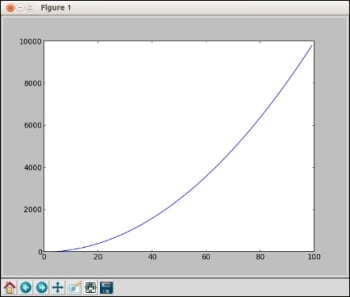The window shows the curve Y = X ** 2 with `X` in the [0, 99] range. As you might have noticed, the window has several icons, some of which are as follows:

•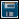: This icon opens a dialog, allowing you to save the graph as a picture file. You can save it as a bitmap picture or a vector picture.
•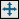: This icon allows you to translate and scale the graphics. Click on it and then move the mouse over the graph. Clicking on the left button of the mouse will translate the graph according to the mouse movements. Clicking on the right button of the mouse will modify the scale of the graphics.
•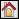: This icon will restore the graph to its initial state, canceling any translation or scaling you might have applied before.

### How it works...

Assuming that you are not very familiar with Python yet, let's analyze the script demonstrated in the previous section.

The first line tells Python that we are using the `matplotlib.pyplot` module. To save on a bit of typing, we make the name `plt` equivalent to `matplotlib.pyplot`. This is a very common practice that you will see in matplotlib code.

The second line creates a list named `X`, with all the integer values from 0 to 99. The range function is used to generate consecutive numbers. You can run the interactive Python interpreter and type the command `range(100)` if you use Python 2, or the command `list(range(100))` if you use Python 3. This will display the list of all the integer values from 0 to 99. In both versions, `sum(range(100))` will compute the sum of the integers from 0 to 99.

The third line creates a list named `Y`, with all the values from the list `X` squared. Building a new list by applying a function to each member of another list is a Python idiom, named list comprehension. The list `Y` will contain the squared values of the list `X` in the same order. So `Y` will contain 0, 1, 4, 9, 16, 25, and so on.

The fourth line plots a curve, where the x coordinates of the curve's points are given in the list `X`, and the y coordinates of the curve's points are given in the list `Y`. Note that the names of the lists can be anything you like.

The last line shows a result, which you will see on the window while running the script.

### There's more...

So what we have learned so far? Unlike plotting packages like gnuplot, matplotlib is not a command interpreter specialized for the purpose of plotting. Unlike Matlab, matplotlib is not an integrated environment for plotting either. matplotlib is a Python module for plotting. Figures are described with Python scripts, relying on a (fairly large) set of functions provided by matplotlib.

Thus, the philosophy behind matplotlib is to take advantage of an existing language, Python. The rationale is that Python is a complete, well-designed, general purpose programming language. Combining matplotlib with other packages does not involve tricks and hacks, just Python code. This is because there are numerous packages for Python for pretty much any task. For instance, to plot data stored in a database, you would use a database package to read the data and feed it to matplotlib. To generate a large batch of statistical graphics, you would use a scientific computing package such as SciPy and Python's I/O modules.

Thus, unlike many plotting packages, matplotlib is very orthogonal—it does plotting and only plotting. If you want to read inputs from a file or do some simple intermediary calculations, you will have to use Python modules and some glue code to make it happen. Fortunately, Python is a very popular language, easy to master and with a large user base. Little by little, we will demonstrate the power of this approach.

## Using NumPy

NumPy is not required to use matplotlib. However, many matplotlib tricks, code samples, and examples use NumPy. A short introduction to NumPy usage will show you the reason.

Along with having Python and matplotlib installed, you also have NumPy installed. You have a text editor and a command terminal.

### How to do it...

Let's plot another curve, `sin(x)`, with x in the [0, 2 * pi] interval. The only difference with the preceding script is the part where we generate the point coordinates. Type and save the following script as `sin-1.py`:

```import math
import matplotlib.pyplot as plt

T = range(100)
X = [(2 * math.pi * t) / len(T) for t in T]
Y = [math.sin(value) for value in X]

plt.plot(X, Y)
plt.show()```

Then, type and save the following script as `sin-2.py`:

```import numpy as np
import matplotlib.pyplot as plt
X = np.linspace(0, 2 * np.pi, 100)
Y = np.sin(X)

plt.plot(X, Y)
plt.show()```

Running either `sin-1.py` or `sin-2.py` will show the following graph exactly: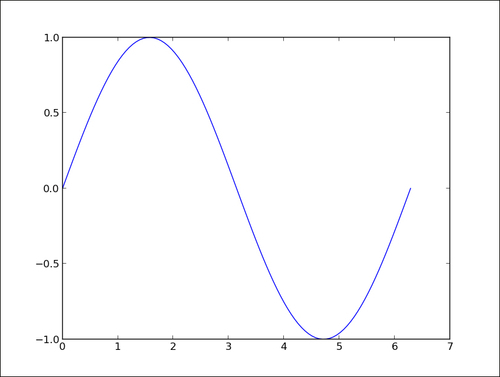### How it works...

The first script, `sin-1.py`, generates the coordinates for a sinusoid using only Python's standard library. The following points describe the steps we performed in the script in the previous section:

1. We created a list `T` with numbers from 0 to 99—our curve will be drawn with 100 points.

2. We computed the x coordinates by simply rescaling the values stored in `T` so that x goes from 0 to 2 pi (the `range()` built-in function can only generate integer values).

3. As in the first example, we generated the y coordinates.

The second script `sin-2.py`, does exactly the same job as `sin-1.py`—the results are identical. However, `sin-2.py` is slightly shorter and easier to read since it uses the NumPy package.

### Tip

NumPy is a Python package for scientific computing. matplotlib can work without NumPy, but using NumPy will save you lots of time and effort. The NumPy package provides a powerful multidimensional array object and a host of functions to manipulate it.

The NumPy package

In `sin-2.py`, the `X` list is now a one-dimensional NumPy array with 100 evenly spaced values between 0 and 2 pi. This is the purpose of the function `numpy.linspace`. This is arguably more convenient than computing as we did in `sin-1.py`. The `Y` list is also a one-dimensional NumPy array whose values are computed from the coordinates of `X`. NumPy functions work on whole arrays as they would work on a single value. Again, there is no need to compute those values explicitly one-by-one, as we did in `sin-1.py`. We have a shorter yet readable code compared to the pure Python version.

### There's more...

NumPy can perform operations on whole arrays at once, saving us much work when generating curve coordinates. Moreover, using NumPy will most likely lead to much faster code than the pure Python equivalent. Easier to read and faster code, what's not to like? The following is an example where we plot the binomial x^2 -2x +1 in the [`-3`,`2`] interval using `200` points:

```import numpy as np
import matplotlib.pyplot as plt

X = np.linspace(-3, 2, 200)
Y = X ** 2 - 2 * X + 1.

plt.plot(X, Y)
plt.show()```

Running the preceding script will give us the result shown in the following graph: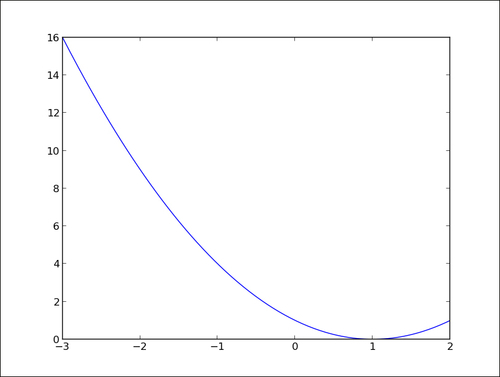Again, we could have done the plotting in pure Python, but it would arguably not be as easy to read. Although matplotlib can be used without NumPy, the two make for a powerful combination.

## Plotting multiple curves

One of the reasons we plot curves is to compare those curves. Are they matching? Where do they match? Where do they not match? Are they correlated? A graph can help to form a quick judgment for more thorough investigations.

### How to do it...

Let's show both `sin(x)` and `cos(x)` in the [0, 2pi] interval as follows:

```import numpy as np
import matplotlib.pyplot as plt

X = np.linspace(0, 2 * np.pi, 100)
Ya = np.sin(X)
Yb = np.cos(X)

plt.plot(X, Ya)
plt.plot(X, Yb)
plt.show()```

The preceding script will give us the result shown in the following graph: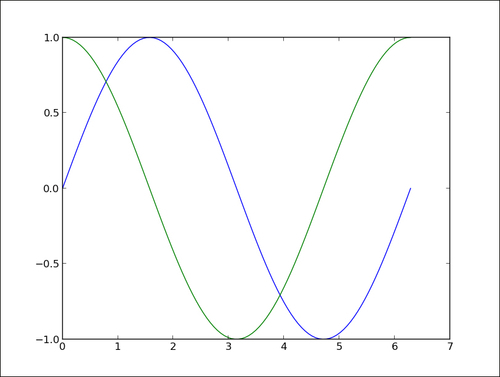### How it works...

The two curves show up with a different color automatically picked up by matplotlib. We use one function `call plt.plot()` for one curve; thus, we have to call `plt.plot()` here twice. However, we still have to call `plt.show()` only once. The functions `calls plt.plot(X, Ya)` and `plt.plot(X, Yb)` can be seen as declarations of intentions. We want to link those two sets of points with a distinct curve for each.

matplotlib will simply keep note of this intention but will not plot anything yet. The `plt.show()` curve, however, will signal that we want to plot what we have described so far.

### There's more...

This deferred rendering mechanism is central to matplotlib. You can declare what you render as and when it suits you. The graph will be rendered only when you call `plt.show()`. To illustrate this, let's look at the following script, which renders a bell-shaped curve, and the slope of that curve for each of its points:

```import numpy as np
import matplotlib.pyplot as plt

def plot_slope(X, Y):
Xs = X[1:] - X[:-1]
Ys = Y[1:] - Y[:-1]
plt.plot(X[1:], Ys / Xs)

X = np.linspace(-3, 3, 100)
Y = np.exp(-X ** 2)

plt.plot(X, Y)
plot_slope(X, Y)

plt.show()```

The preceding script will produce the following graph: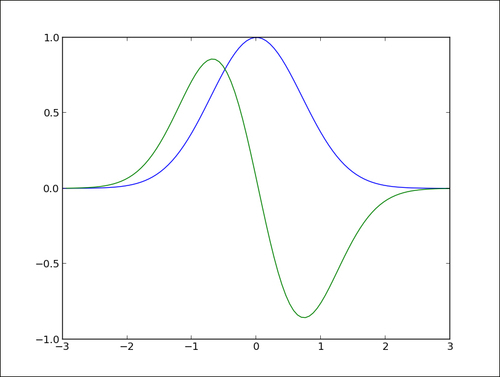One of the function call, `plt.plot()`, is done inside the `plot_slope` function, which does not have any influence on the rendering of the graph as `plt.plot()` simply declares what we want to render, but does not execute the rendering yet. This is very useful when writing scripts for complex graphics with a lot of curves. You can use all the features of a proper programming language—loop, function calls, and so on— to compose a graph.

## Plotting curves from file data

As explained earlier, matplotlib only handles plotting. If you want to plot data stored in a file, you will have to use Python code to read the file and extract the data you need.

### How to do it...

Let's assume that we have time series stored in a plain text file named `my_data.txt` as follows:

```0  0
1  1
2  4
4 16
5 25
6 36```

A minimalistic pure Python approach to read and plot that data would go as follows:

```import matplotlib.pyplot as plt

X, Y = [], []
for line in open('my_data.txt', 'r'):
values = [float(s) for s in line.split()]
X.append(values)
Y.append(values)

plt.plot(X, Y)
plt.show()```

This script, together with the data stored in `my_data.txt`, will produce the following graph: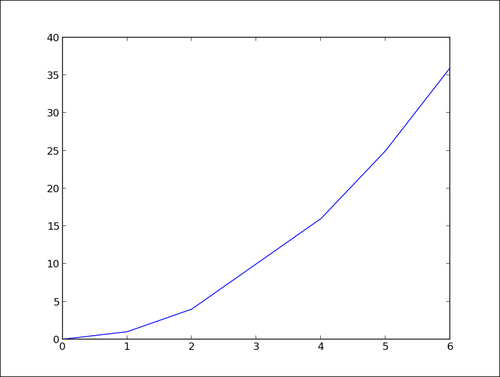### How it works...

The following are some explanations on how the preceding script works:

• The line `X, Y = [], []` initializes the list of coordinates `X` and `Y` as empty lists.

• The line `for line in open('my_data.txt', 'r')` defines a loop that will iterate each line of the text file `my_data.txt`. On each iteration, the current line extracted from the text file is stored as a string in the variable line.

• The line `values = [float(s) for s in line.split()]` splits the current line around empty characters to form a string of tokens. Those tokens are then interpreted as floating point values. Those values are stored in the list values.

• Then, in the two next lines, `X.append(values)` and `Y.append(values)`, the values stored in `values` are appended to the lists `X` and `Y`.

The following equivalent one-liner to read a text file may bring a smile to those more familiar with Python:

```import matplotlib.pyplot as plt

with open('my_data.txt', 'r') as f:
X, Y = zip(*[[float(s) for s in line.split()] for line in f])

plt.plot(X, Y)
plt.show()```

### There's more...

In our data loading code, note that there is no serious checking or error handling going on. In any case, one might remember that a good programmer is a lazy programmer. Indeed, since NumPy is so often used with matplotlib, why not use it here? Run the following script to enable NumPy:

```import numpy as np
import matplotlib.pyplot as plt

plt.plot(data[:,0], data[:,1])
plt.show()```

This is as short as the one-liner shown in the preceding section, yet easier to read, and it will handle many error cases that our pure Python code does not handle. The following point describes the preceding script:

• The `numpy.loadtxt()` function reads a text file and returns a 2D array. With NumPy, 2D arrays are not a list of lists, they are true, full-blown matrices.

• The variable `data` is a NumPy 2D array, which give us the benefit of being able to manipulate rows and columns of a matrix as a 1D array. Indeed, in the line `plt.plot(data[:,0], data[:,1])`, we give the first column of data as x coordinates and the second column of data as y coordinates. This notation is specific to NumPy.

Along with making the code shorter and simpler, using NumPy brings additional advantages. For large files, using NumPy will be noticeably faster (the NumPy module is mostly written in C), and storing the whole dataset as a NumPy array can save memory as well. Finally, using NumPy allows you to support other common file formats (CVS and Matlab) for numerical data without much effort.

As a way to demonstrate all that we have seen so far, let's consider the following task. A file contains N columns of values, describing N–1 curves. The first column contains the x coordinates, the second column contains the y coordinates of the first curve, the third column contains the y coordinates of the second curve, and so on. We want to display those N–1 curves. We will do so by using the following code:

```import numpy as np
import matplotlib.pyplot as plt

for column in data.T:
plt.plot(data[:,0], column)

plt.show()```

The file `my_data.txt` should contain the following content:

```0 0 6
1 1 5
2 4 4
4 16 3
5 25 2
6 36 1```

Then we get the following graph: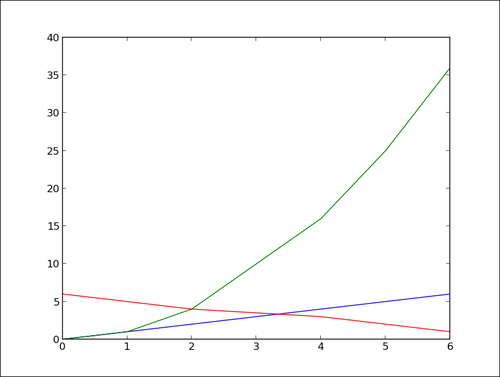We did the job with little effort by exploiting two tricks. In NumPy notation, `data.T` is a transposed view of the 2D array data—rows are seen as columns and columns are seen as rows. Also, we can iterate over the rows of a multidimensional array by doing `for row in data`. Thus, doing `for column in` `data.T` will iterate over the columns of an array. With a few lines of code, we have a fairly general plotting generic script.

## Plotting points

When displaying a curve, we implicitly assume that one point follows another—our data is the time series. Of course, this does not always have to be the case. One point of the data can be independent from the other. A simple way to represent such kind of data is to simply show the points without linking them.

### How to do it...

The following script displays 1024 points whose coordinates are drawn randomly from the [0,1] interval:

```import numpy as np
import matplotlib.pyplot as plt

data = np.random.rand(1024, 2)

plt.scatter(data[:,0], data[:,1])
plt.show()```

The preceding script will produce the following graph: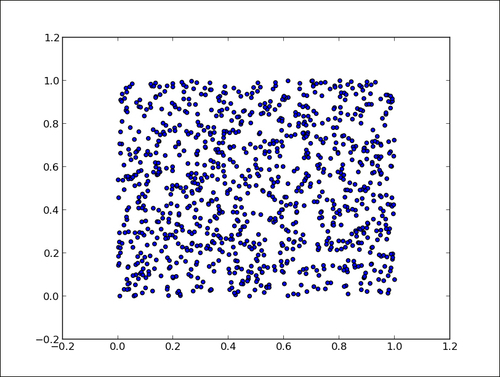### How it works...

The function `plt.scatter()` works exactly like `plt.plot()`, taking the x and y coordinates of points as input parameters. However, each point is simply shown with one marker. Don't be fooled by this simplicity—`plt.scatter()` is a rich command. By playing with its many optional parameters, we can achieve many different effects. We will cover this in Chapter 2, Customizing the Color and Styles, and Chapter 3, Working with Annotations.

## Plotting bar charts

Bar charts are a common staple of plotting package, and even matplotlib has them.

### How to do it...

The dedicated function for bar charts is `pyplot.bar()`. We will enable this function by executing the following script:

```import matplotlib.pyplot as plt

data = [5., 25., 50., 20.]

plt.bar(range(len(data)), data)
plt.show()```

The preceding script will produce the following graph: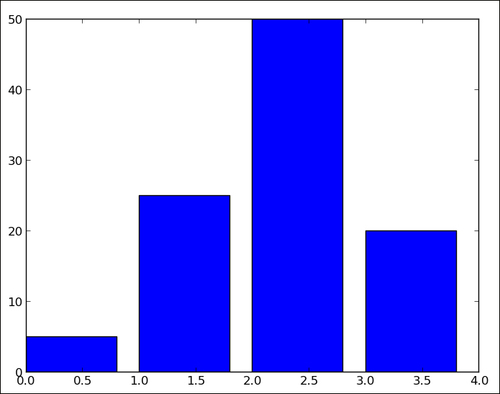### How it works...

For each value in the list data, one vertical bar is shown. The `pyplot.bar()` function receives two arguments—the x coordinate for each bar and the height of each bar. Here, we use the coordinates 0, 1, 2, and so on, for each bar, which is the purpose of `range(len(data))`.

### There's more...

Through an optional parameter, `pyplot.bar()` provides a way to control the bar's thickness. Moreover, we can also obtain horizontal bars using the twin brother of `pyplot.bar()`, that is, `pyplot.barh()`.

#### The thickness of a bar

By default, a bar will have a thickness of 0.8 units. Because we put a bar at each unit length, we have a gap of 0.2 between them. You can, of course, fiddle with this thickness parameter. For instance, by setting it to 1:

```import matplotlib.pyplot as plt

data = [5., 25., 50., 20.]

plt.bar(range(len(data)), data, width = 1.)
plt.show()```

The preceding minimalistic script will produce the following graph: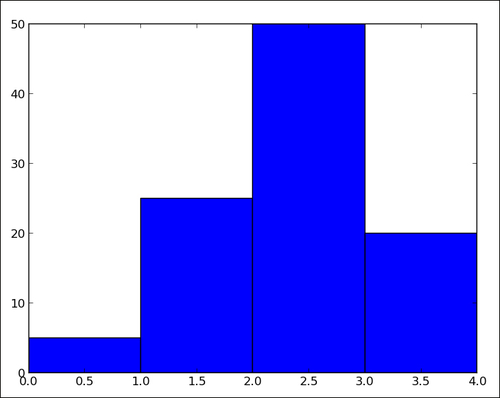Now, the bars have no gap between them. The matplotlib bar chart function `pyplot.bar()` will not handle the positioning and thickness of the bars. The programmer is in charge. This flexibility allows you to create many variations on bar charts.

#### Horizontal bars

If you are more into horizontal bars, use the `barh()` function, which is the strict equivalent of `bar()`, apart from giving horizontal rather than vertical bars:

```import matplotlib.pyplot as plt

data = [5., 25., 50., 20.]

plt.barh(range(len(data)), data)
plt.show()```

The preceding script will produce the following graph: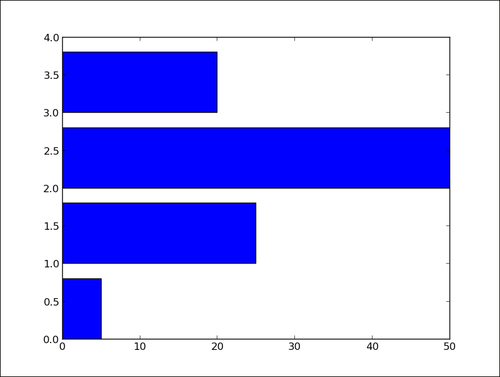## Plotting multiple bar charts

When comparing several quantities and when changing one variable, we might want a bar chart where we have bars of one color for one quantity value.

### How to do it...

We can plot multiple bar charts by playing with the thickness and the positions of the bars as follows:

```import numpy as np
import matplotlib.pyplot as plt

data = [[5., 25., 50., 20.],
[4., 23., 51., 17.],
[6., 22., 52., 19.]]

X = np.arange(4)
plt.bar(X + 0.00, data, color = 'b', width = 0.25)
plt.bar(X + 0.25, data, color = 'g', width = 0.25)
plt.bar(X + 0.50, data, color = 'r', width = 0.25)

plt.show()```

The preceding script will produce the following graph: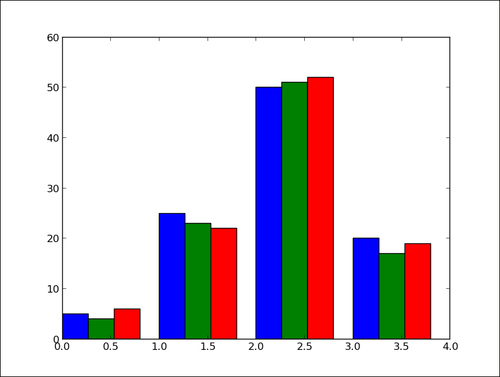### How it works...

The `data` variable contains three series of four values. The preceding script will show three bar charts of four bars. The bars will have a thickness of 0.25 units. Each bar chart will be shifted 0.25 units from the previous one. Color has been added for clarity. This topic will be detailed in Chapter 2, Customizing the Color and Styles.

### There's more...

The code shown in the preceding section is quite tedious as we repeat ourselves by shifting the three bar charts manually. We can do this better by using the following code:

```import numpy as np
import matplotlib.pyplot as plt

data = [[5., 25., 50., 20.],
[4., 23., 51., 17.],
[6., 22., 52., 19.]]

color_list = ['b', 'g', 'r']
gap = .8 / len(data)
for i, row in enumerate(data):
X = np.arange(len(row))
plt.bar(X + i * gap, row,
width = gap,
color = color_list[i % len(color_list)])

plt.show()```

Here, we iterate over each row of data with the loop `for i, row in enumerate(data)`. The iterator `enumerate` returns both the current row and its index. Generating the position of each bar for one bar chart is done with a list comprehension. This script will produce the same result as the previous script, but would not require any change if we add rows or columns of data.

## Plotting stacked bar charts

Stacked bar charts are of course possible by using a special parameter from the `pyplot.bar()` function.

### How to do it...

The following script stacks two bar charts on each other:

```import matplotlib.pyplot as plt

A = [5., 30., 45., 22.]
B = [5., 25., 50., 20.]

X = range(4)

plt.bar(X, A, color = 'b')
plt.bar(X, B, color = 'r', bottom = A)
plt.show()```

The preceding script will produce the following graph: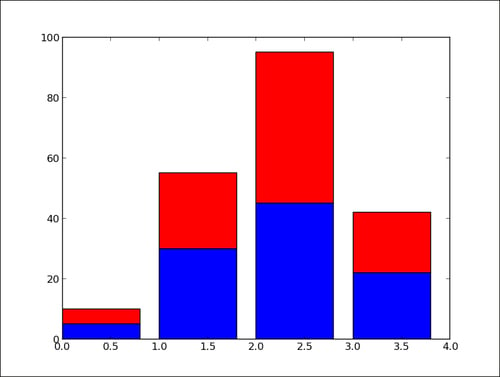### How it works...

The optional `bottom` parameter of the `pyplot.bar()` function allows you to specify a starting value for a bar. Instead of running from zero to a value, it will go from the bottom to value. The first call to `pyplot.bar()` plots the blue bars. The second call to `pyplot.bar()` plots the red bars, with the bottom of the red bars being at the top of the blue bars.

### There's more...

When stacking more than two set of values, the code gets less pretty as follows:

```import numpy as np
import matplotlib.pyplot as plt

A = np.array([5., 30., 45., 22.])
B = np.array([5., 25., 50., 20.])
C = np.array([1.,  2.,  1.,  1.])
X = np.arange(4)

plt.bar(X, A, color = 'b')
plt.bar(X, B, color = 'g', bottom = A)
plt.bar(X, C, color = 'r', bottom = A + B)

plt.show()```

For the third bar chart, we have to compute the bottom values as `A + B`, the coefficient-wise sum of A and B. Using NumPy helps to keep the code compact but readable. This code is, however, fairly repetitive and works for only three stacked bar charts. We can do better using the following code:

```import numpy as np
import matplotlib.pyplot as plt

data = np.array([[5., 30., 45., 22.],
[5., 25., 50., 20.],
[1.,  2.,  1.,  1.]]

color_list = ['b', 'g', 'r']

X = np.arange(data.shape)
for i in range(data.shape):
plt.bar(X, data[i],
bottom = np.sum(data[:i], axis = 0),
color = color_list[i % len(color_list)])

plt.show()```

Here, we store the data in a NumPy array, one row for one bar chart. We iterate over each row of data. For the ith row, the `bottom` parameter receives the sum of all the rows before the ith row. Writing the script this way, we can stack as many bar charts as we wish with minimal effort when changing the input data.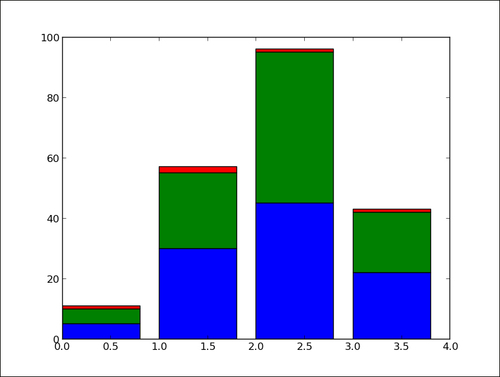## Plotting back-to-back bar charts

A simple but useful trick is to display two bar charts back-to-back at the same time. Think of an age pyramid of a population, showing the number of people within different age ranges. On the left side, we show the male population, while on the right we show the female population.

### How to do it...

The idea is to have two bar charts, using a simple trick, that is, the length/height of one bar can be negative!

```import numpy as np
import matplotlib.pyplot as plt

women_pop = np.array([5., 30., 45., 22.])
men_pop     = np.array( [5., 25., 50., 20.])
X = np.arange(4)

plt.barh(X, women_pop, color = 'r')
plt.barh(X, -men_pop, color = 'b')
plt.show()```

The preceding script will produce the following graph: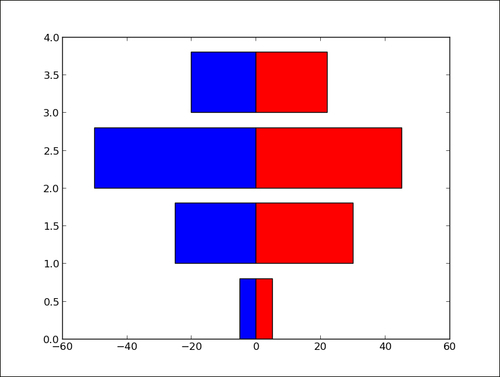### How it works...

The bar chart for the female population (in red) is plotted as usual. However, the bar chart for the male population (in blue) has its bar extending to the left rather than the right. Indeed, the lengths of the bars for the blue bar chart are negative values. Rather than editing the input values, we use a list comprehension to negate values for the male population bar chart.

## Plotting pie charts

To compare the relative importance of quantities, nothing like a good old pie—pie chart, that is.

### How to do it...

The dedicated pie-plotting function `pyplot.pie()` will do the job. We will use this function in the following code:

```import matplotlib.pyplot as plt

data = [5, 25, 50, 20]

plt.pie(data)
plt.show()```

The preceding simple script will display the following pie diagram: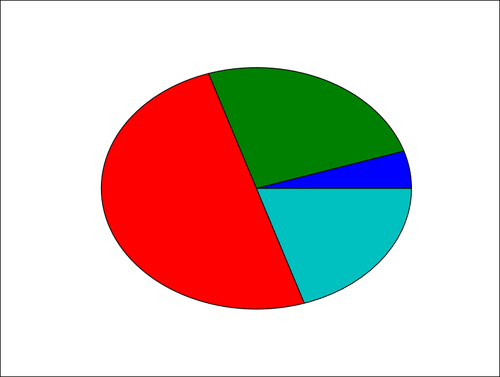### How it works...

The `pyplot.pie()` function simply takes a list of values as the input. Note that the input data is a list; it could be a NumPy array. You do not have to adjust the data so that it adds up to 1 or 100. You just have to give values to matplolib and it will automatically compute the relative areas of the pie chart.

## Plotting histograms

Histograms are graphical representations of a probability distribution. In fact, a histogram is just a specific kind of a bar chart. We could easily use matplotlib's bar chart function and do some statistics to generate histograms. However, histograms are so useful that matplotlib provides a function just for them. In this recipe, we are going to see how to use this histogram function.

### How to do it...

The following script draws `1000` values from a normal distribution and then generates histograms with 20 bins:

```import numpy as np
import matplotlib.pyplot as plt

X = np.random.randn(1000)

plt.hist(X, bins = 20)
plt.show()```

The histogram will change a bit each time we run the script as the dataset is randomly generated. The preceding script will display the following graph: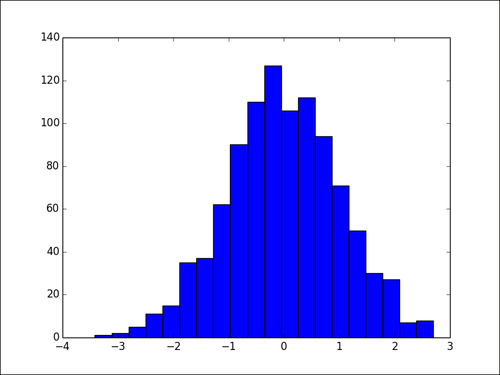### How it works...

The `pyplot.hist()` function takes a list of values as the input. The range of the values will be divided into equal-sized bins (10 bins by default). The `pyplot.hist()` function will generate a bar chart, one bar for one bin. The height of one bar is the number of values following in the corresponding bin. The number of bins is determined by the optional parameter bins. By setting the optional parameter `normed` to `True`, the bar height is normalized and the sum of all bar heights is equal to 1.

## Plotting boxplots

Boxplot allows you to compare distributions of values by conveniently showing the median, quartiles, maximum, and minimum of a set of values.

### How to do it...

The following script shows a boxplot for 100 random values drawn from a normal distribution:

```import numpy as np
import matplotlib.pyplot as plt

data = np.random.randn(100)

plt.boxplot(data)
plt.show()```

A boxplot will appear that represents the samples we drew from the random distribution. Since the code uses a randomly generated dataset, the resulting figure will change slightly every time the script is run.

The preceding script will display the following graph: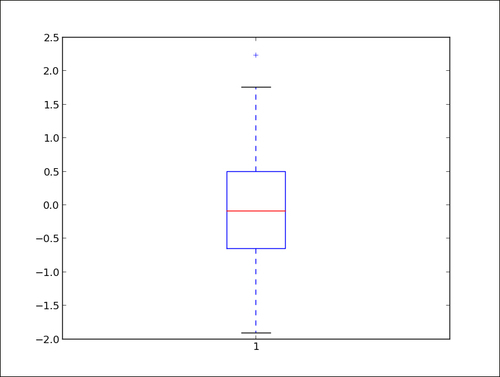### How it works...

The `data = [random.gauss(0., 1.) for i in range(100)]` variable generates 100 values drawn from a normal distribution. For demonstration purposes, such values are typically read from a file or computed from other data. The `plot.boxplot()` function takes a set of values and computes the mean, median, and other statistical quantities on its own. The following points describe the preceding boxplot:

• The red bar is the median of the distribution.

• The blue box includes 50 percent of the data from the lower quartile to the upper quartile. Thus, the box is centered on the median of the data.

• The lower whisker extends to the lowest value within 1.5 IQR from the lower quartile.

• The upper whisker extends to the highest value within 1.5 IQR from the upper quartile.

• Values further from the whiskers are shown with a cross marker.

### There's more...

To show more than one boxplot in a single graph, calling `pyplot.boxplot()` once for each boxplot is not going to work. It will simply draw the boxplots over each other, making a messy, unreadable graph. However, we can draw several boxplots with just one single call to `pyplot.boxplot()` as follows:

```import numpy as np
import matplotlib.pyplot as plt

data = np.random.randn(100, 5)

plt.boxplot(data)
plt.show()```

The preceding script displays the following graph: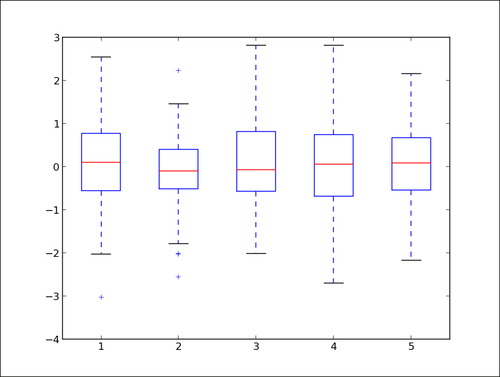The `pyplot.boxplot()` function accepts a list of lists as the input, rendering a boxplot for each sublist.

## Plotting triangulations

Triangulations arise when dealing with spatial locations. Apart from showing distances between points and neighborhood relationships, triangulation plots can be a convenient way to represent maps. matplotlib provides a fair amount of support for triangulations.

### How to do it...

As in the preceding examples, the following few lines of code are enough:

```import numpy as np
import matplotlib.pyplot as plt
import matplotlib.tri as tri

data = np.random.rand(100, 2)

triangles = tri.Triangulation(data[:,0], data[:,1])

plt.triplot(triangles)
plt.show()```

Every time the script is run, you will see a different triangulation as the cloud of points that is triangulated is generated randomly.

The preceding script displays the following graph: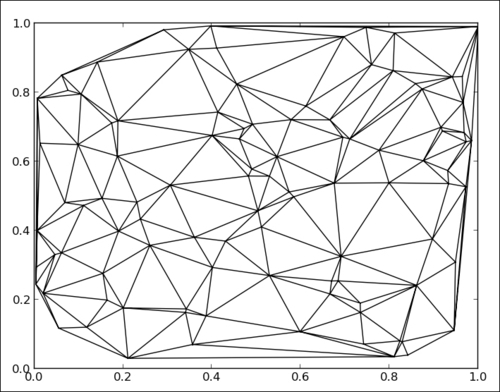### How it works...

We import the `matplotlib.tri` module, which provides helper functions to compute triangulations from points. In this example, for demonstration purpose, we generate a random cloud of points using the following code:

`data = np.random.rand(100, 2)`

We compute a triangulation and store it in the triangles' variable with the help of the following code:

`triangles = tri.Triangulation(data[:,0], data[:,1])`

The `pyplot.triplot()` function simply takes triangles as inputs and displays the triangulation result.